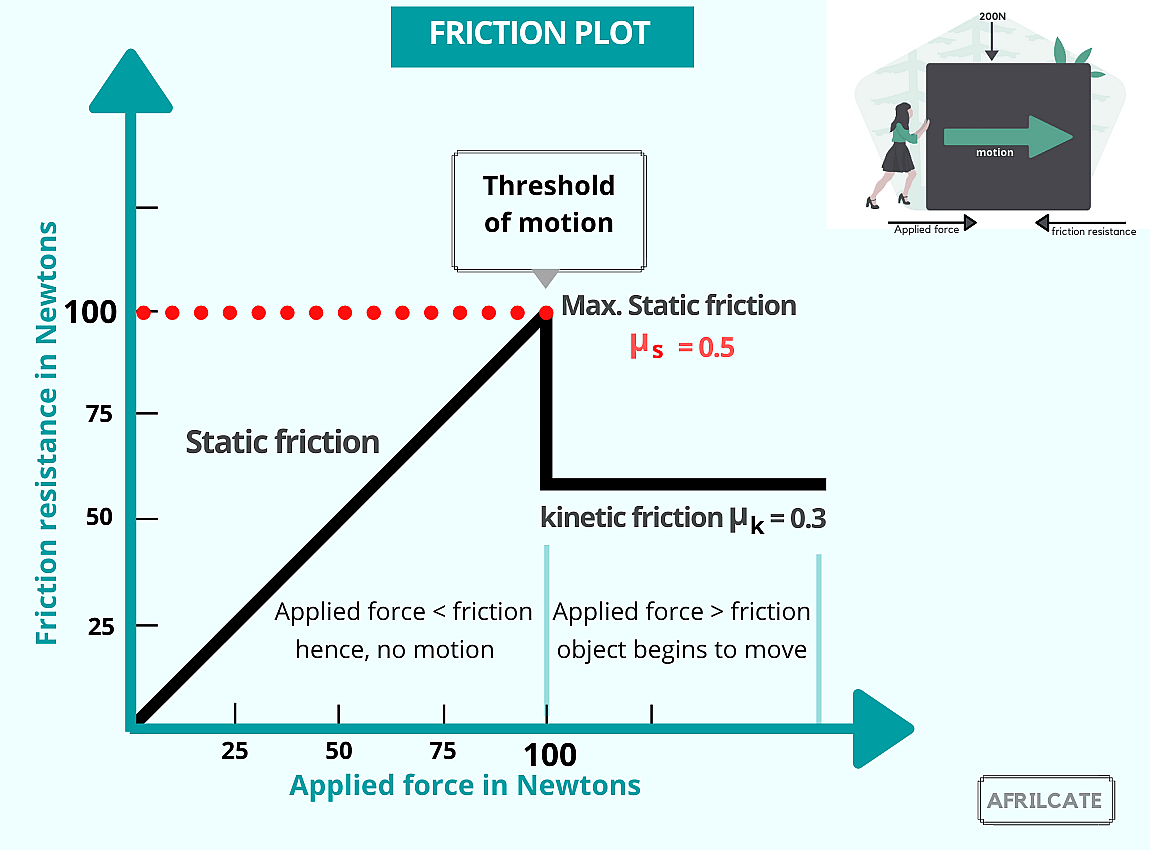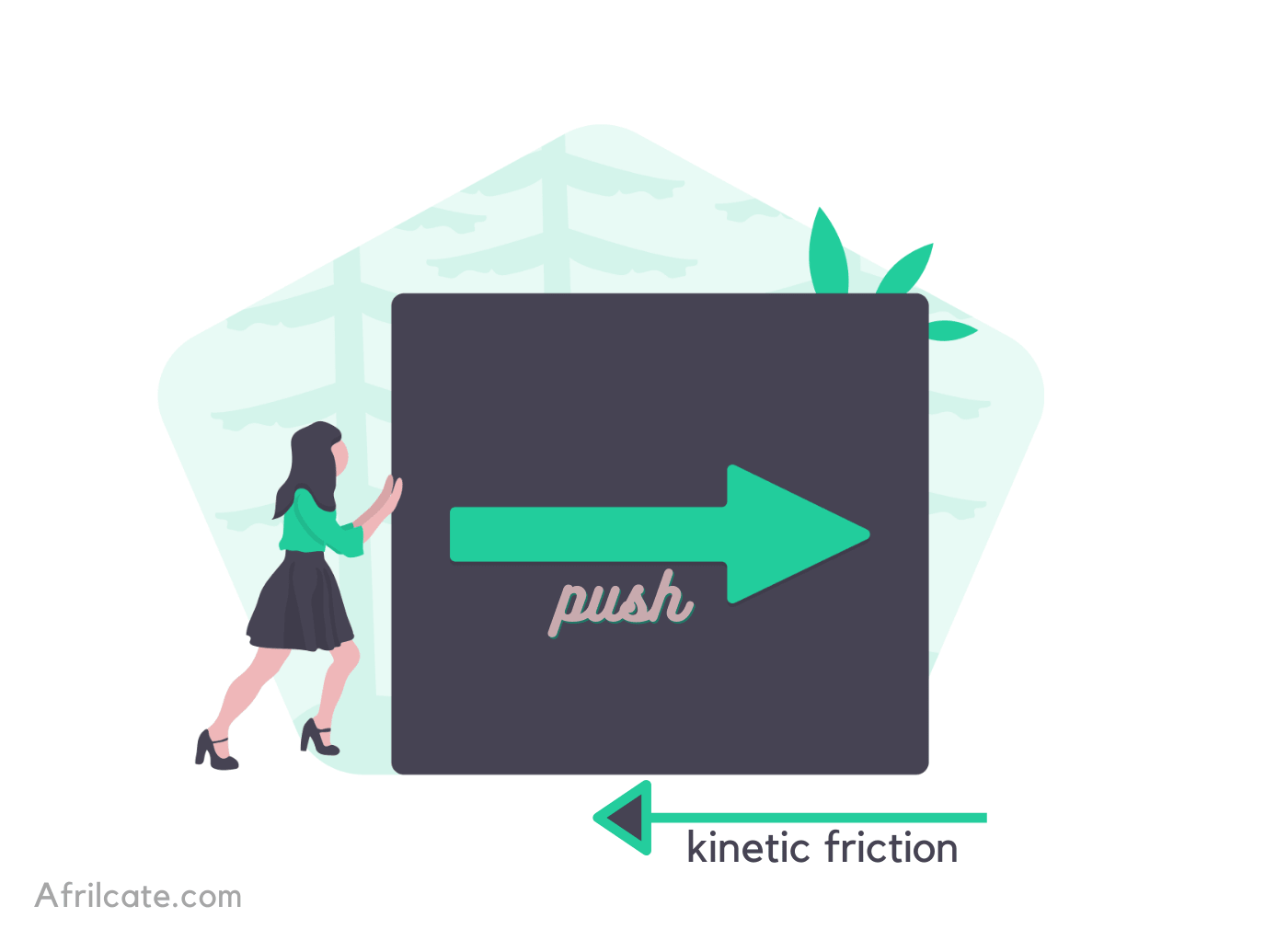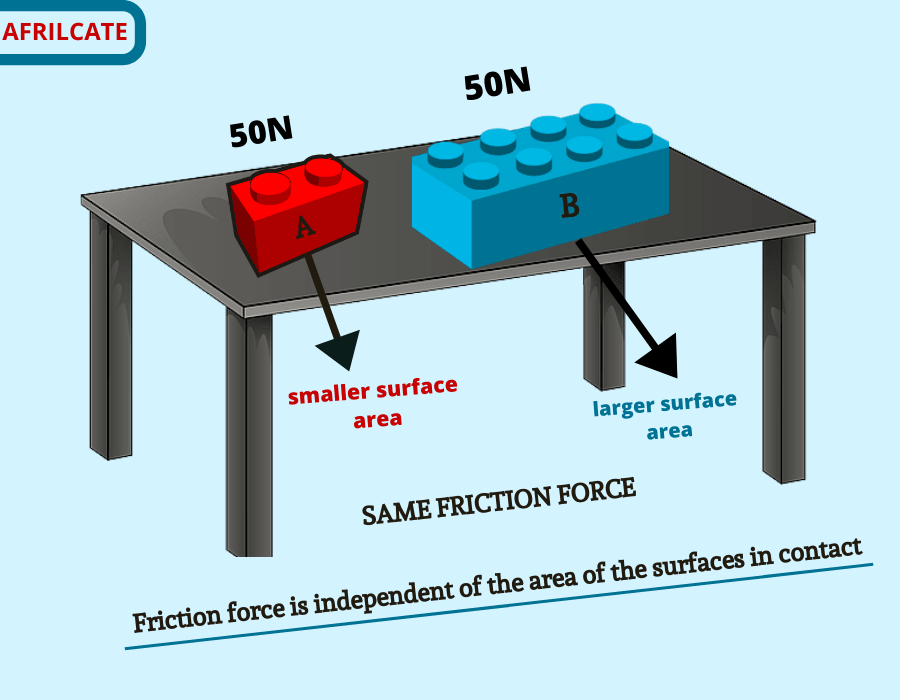Select Page

# The 5 Laws of Friction (with Detailed Explanation)

updated Oct 13, 2020### Table of Content

1. Laws of Friction

# Laws of Friction

1. The maximum static frictional force (limiting friction) is directly proportional to the normal force.
2. Friction acts in a direction opposite to the direction of motion.
3. The frictional force is independent of the area of contact surfaces.
4. Frictional force depends on the nature of the surfaces in contact.
5. Kinetic friction is independent of the sliding velocity.

# Overview of Friction

Friction is the resistance between contacting bodies when one moves relative to another.

It’s applicable in every sphere of life and this makes it one of the fundamental topics in physics.

There are three major types of friction.

1. Static friction: friction force between an object at rest relative to a surface.
2. Kinetic friction: friction force between surfaces that moves or slides relative to each other.
3. Rolling friction: friction force that resists the rolling motion of an object against a surface.

# Detailed Explanation of Friction Laws

1. The maximum static frictional force (limiting friction) is directly proportional to the normal force.

Fstatic α N

The maximum static frictional force is also known as the limiting friction.

This law simply tells us that as the normal reaction increases, the frictional force will also increase.

The normal reaction depends on the weight of the object.

This law of friction is known as the Amontons’  First Law.1. Friction acts in a direction opposite to the direction of motion.

This law correlates with the definition of friction.

Since friction is a resisting force it will always act opposite to the direction of push or pull.1. The frictional force is independent of the area of contact surfaces.

consider these two Lego bricks of the same weight sitting on a wooden table.

1. The frictional force is independent of the area of contact surfaces.

consider these two Lego bricks of the same weight sitting on a wooden table.One would think that block B will have a higher friction force because of its larger surface contact but that’s not true.

Do you know why?

Recall that pressure equals force divided by area of contact. P = F/A

For objects with the same mass, it means that the increase in the contact area is offset by a corresponding reduction in pressure.

The frictional force will therefore remain unchanged irrespective of the contact area of the object.

1. Frictional force depends on the nature of the surfaces in contact.

The nature of the surfaces in contact affects the coefficient of friction.

Some surfaces like higher friction coefficient like rubber on pavements.

while others have a lower friction coefficient just like ice on steel.

Keep in mind that the coefficient of friction is the constant of proportionality in the friction formula.

Fstatic α N

Fstatic = µfrictionN

Where µ = coefficient of friction

The surface nature varies based on certain factors like:

• specific material property,
• smoothness or roughness of contact surface,
• dryness or wetness of contact surface.

This particular friction law is also known as the Amontons’ second Law.

1. Kinetic friction is independent of the sliding velocity.

Also known as the Coulomb’s Law of Friction, it says that the kinetic friction exerted between the contact surface of two dry solids is not affected by the magnitude of the relative surface velocity.

Kinetic friction depends on factors like:

• the kinetic coefficient of friction and
• normal force

It pays no attention to the relative speed between the contact area.

### What are your thoughts on the laws of friction?

Have you come across any conflicting or contradicting statements in the past?

Share your thoughts and answers with us in the comment section below.